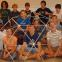Java Games: Flashcards, matching, concentration, and word search.# PC Log Equations Flashcards

Pre Calculus Chapter 3 Logarithmic and Exponental EQUATIONS

AB
Common Loglog (the base is understood to be 10)
Natural Logln (the base is understood to be e)
Exponential Form:,Logarithmic Form:,Exponential Form:,Logarithmic Form:,Mrs. Wolfe Mathematics Teacher Carlisle High School Carlisle, PA

 This activity was created by a Quia Web subscriber. Learn more about QuiaCreate your own activities